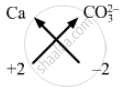# Work Out the Formulae for the Following Compounds: Calcium Carbonate - Science

Short Note

Work out the formulae for the following compounds:

Calcium carbonate

#### Solution

Valency of Calcium = 2
Valency of Carbonate ion = −2Formula for calcium carbonate can be worked out by crossing  the valencies of the constituent elements/ions. +2 positive charge of calcium neutralizes the −2 negative charge of carbonate ion.
Therefore, formula for calcium carbonate is: CaCO3.

Concept: Laws of Chemical Combination - Law of Constant Proportions or Law of Definite Proportions
Is there an error in this question or solution?

#### APPEARS IN

Lakhmir Singh Class 9 Chemistry - Science Part 2
Chapter 3 Atoms and Molecules
Short Answers | Q 20.2 | Page 151